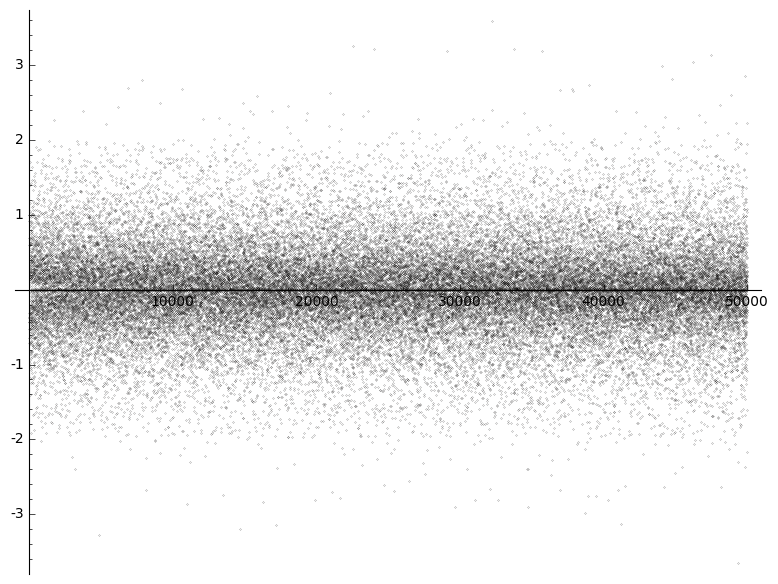Tag Archives: dirichlet series

A Notebook Preparing for a Talk at Quebec-Maine

This is a notebook containing a representative sample of the code I used to  generate the results and pictures presented at the Quebec-Maine Number Theory Conference on 9 October 2016. It was written in a Jupyter Notebook using Sage 7.3, and later converted for presentation on this site.
There is a version of the notebook available on github. Alternately, a static html version without WordPress formatting is available here. Finally, this notebook is also available in pdf form.
The slides for my talk are available here.

Testing for a Generalized Conjecture on Iterated Sums of Coefficients of Cusp Forms¶

Let $f$ be a weight $k$ cusp form with Fourier expansion

$$f(z) = \sum_{n \geq 1} a(n) e(nz).$$

Deligne has shown that $a(n) \ll n^{\frac{k-1}{2} + \epsilon}$. It is conjectured that

$$S_f^1(n) := \sum_{m \leq X} a(m) \ll X^{\frac{k-1}{2} + \frac{1}{4} + \epsilon}.$$

It is known that this holds on average, and we recently showed that this holds on average in short intervals.
(See HKLDW1, HKLDW2, and HKLDW3 for details and an overview of work in this area).
This is particularly notable, as the resulting exponent is only 1/4 higher than that of a single coefficient.
This indicates extreme cancellation, far more than what is implied merely by the signs of $a(n)$ being random.

It seems that we also have

$$\sum_{m \leq X} S_f^1(m) \ll X^{\frac{k-1}{2} + \frac{2}{4} + \epsilon}.$$

That is, the sum of sums seems to add in only an additional 1/4 exponent.
This is unexpected and a bit mysterious.

The purpose of this notebook is to explore this and higher conjectures.
Define the $j$th iterated sum as

$$S_f^j(X) := \sum_{m \leq X} S_f^{j-1} (m).$$

Then we numerically estimate bounds on the exponent $\delta(j)$ such that

$$S_f^j(X) \ll X^{\frac{k-1}{2} + \delta(j) + \epsilon}.$$

In :
# This was written in SageMath 7.3 through a Jupyter Notebook.

# sage plays strangely with ipython. This re-allows inline plotting
from IPython.display import display, Image


We first need a list of coefficients of one (or more) cusp forms.
For initial investigation, we begin with a list of 50,000 coefficients of the weight $12$ cusp form on $\text{SL}(2, \mathbb{Z})$, $\Delta(z)$, i.e. Ramanujan’s delta function.
We will use the data associated to the 50,000 coefficients for pictoral investigation as well.

We will be performing some numerical investigation as well.
For this, we will use the first 2.5 million coefficients of $\Delta(z)$

In :
# Gather 10 coefficients for simple checking
check_10 = delta_qexp(11).coefficients()
print check_10

fiftyk_coeffs = delta_qexp(50000).coefficients()
print fiftyk_coeffs[:10] # these match expected

twomil_coeffs = delta_qexp(2500000).coefficients()
print twomil_coeffs[:10] # these also match expected

[1, -24, 252, -1472, 4830, -6048, -16744, 84480, -113643, -115920]
[1, -24, 252, -1472, 4830, -6048, -16744, 84480, -113643, -115920]
[1, -24, 252, -1472, 4830, -6048, -16744, 84480, -113643, -115920]

In :
# Function which iterates partial sums from a list of coefficients

def partial_sum(baselist):
ret_list = [baselist]
for b in baselist[1:]:
ret_list.append(ret_list[-1] + b)
return ret_list

print check_10
print partial_sum(check_10) # Should be the partial sums

[1, -24, 252, -1472, 4830, -6048, -16744, 84480, -113643, -115920]
[1, -23, 229, -1243, 3587, -2461, -19205, 65275, -48368, -164288]

In :
# Calculate the first 10 iterated partial sums
# We store them in a single list list, sums_list
# the zeroth elelemnt of the list is the array of initial coefficients
# the first element is the array of first partial sums, S_f(n)
# the second element is the array of second iterated partial sums, S_f^2(n)

fiftyk_sums_list = []
fiftyk_sums_list.append(fiftyk_coeffs) # zeroth index contains coefficients
for j in range(10):                    # jth index contains jth iterate
fiftyk_sums_list.append(partial_sum(fiftyk_sums_list[-1]))

print partial_sum(check_10)
print fiftyk_sums_list[:10]         # should match above

twomil_sums_list = []
twomil_sums_list.append(twomil_coeffs) # zeroth index contains coefficients
for j in range(10):                    # jth index contains jth iterate
twomil_sums_list.append(partial_sum(twomil_sums_list[-1]))

print twomil_sums_list[:10]         # should match above

[1, -23, 229, -1243, 3587, -2461, -19205, 65275, -48368, -164288]
[1, -23, 229, -1243, 3587, -2461, -19205, 65275, -48368, -164288]
[1, -23, 229, -1243, 3587, -2461, -19205, 65275, -48368, -164288]


As is easily visible, the sums alternate in sign very rapidly.
For instance, we believe tha the first partial sums should change sign about once every $X^{1/4}$ terms in the interval $[X, 2X]$.
In this exploration, we are interested in the sizes of the coefficients.
But in HKLDW3, we investigated some of the sign changes of the partial sums.

Now seems like a nice time to briefly look at the data we currently have.
What do the first 50 thousand coefficients look like?
So we normalize them, getting $A(n) = a(n)/n^{5.5}$ and plot these coefficients.

In :
norm_list = []
for n,e in enumerate(fiftyk_coeffs, 1):
normalized_element = 1.0 * e / (1.0 * n**(5.5))
norm_list.append(normalized_element)
print norm_list[:10]

1

In :
# Make a quick display
normed_coeffs_plot = scatter_plot(zip(range(1,60000), norm_list), markersize=.02)
normed_coeffs_plot.save("normed_coeffs_plot.png")
display(Image("normed_coeffs_plot.png"))Since some figures will be featuring prominently in the talk I’m giving at Quebec-Maine, let us make high-quality figures now.

1. 00000000000000, -0.530330085889911, 0.598733612492945, -0.718750000000000, 0.691213333204735, -0.317526448138560, -0.376547696558964, 0.911504835123284, -0.641518061271148, -0.366571226366719
Posted in Math.NT, Mathematics, Open, Programming, sagemath | | 1 Comment